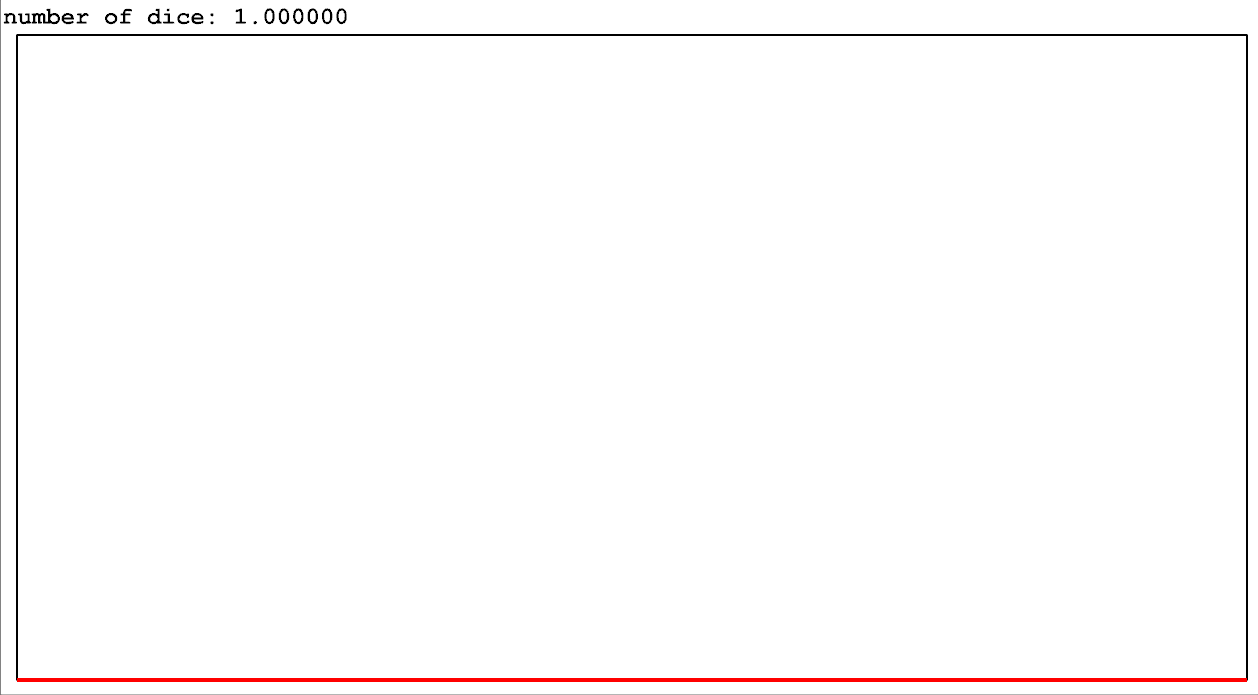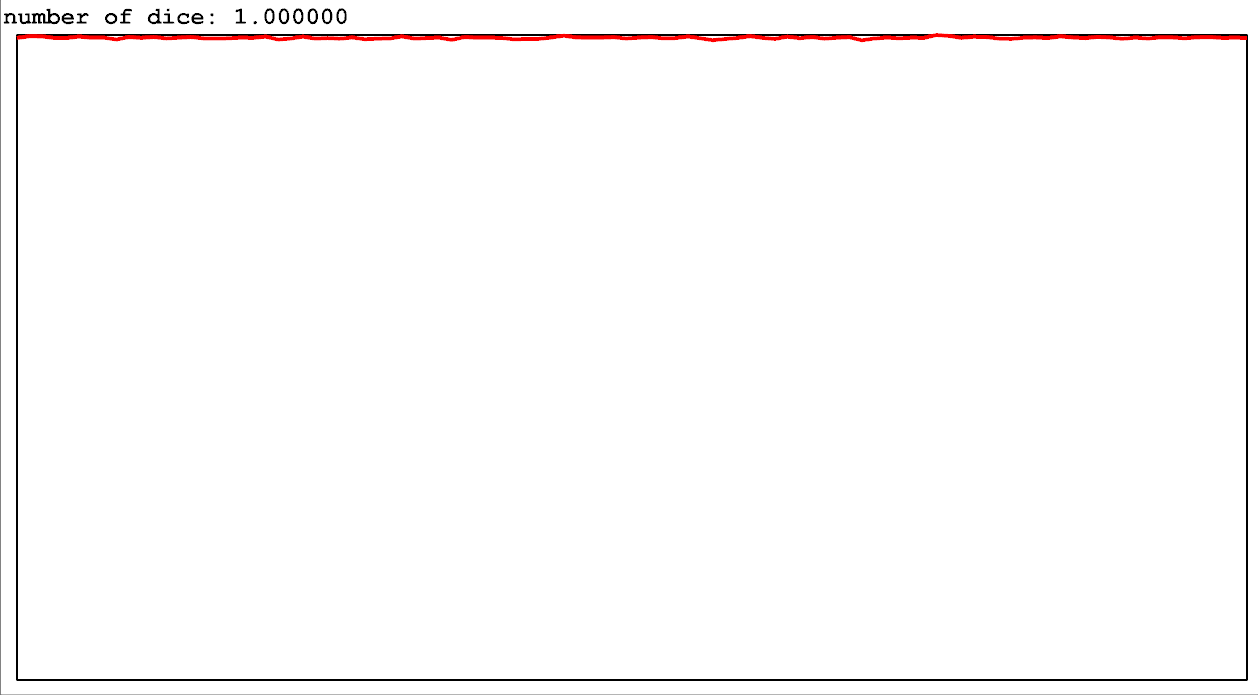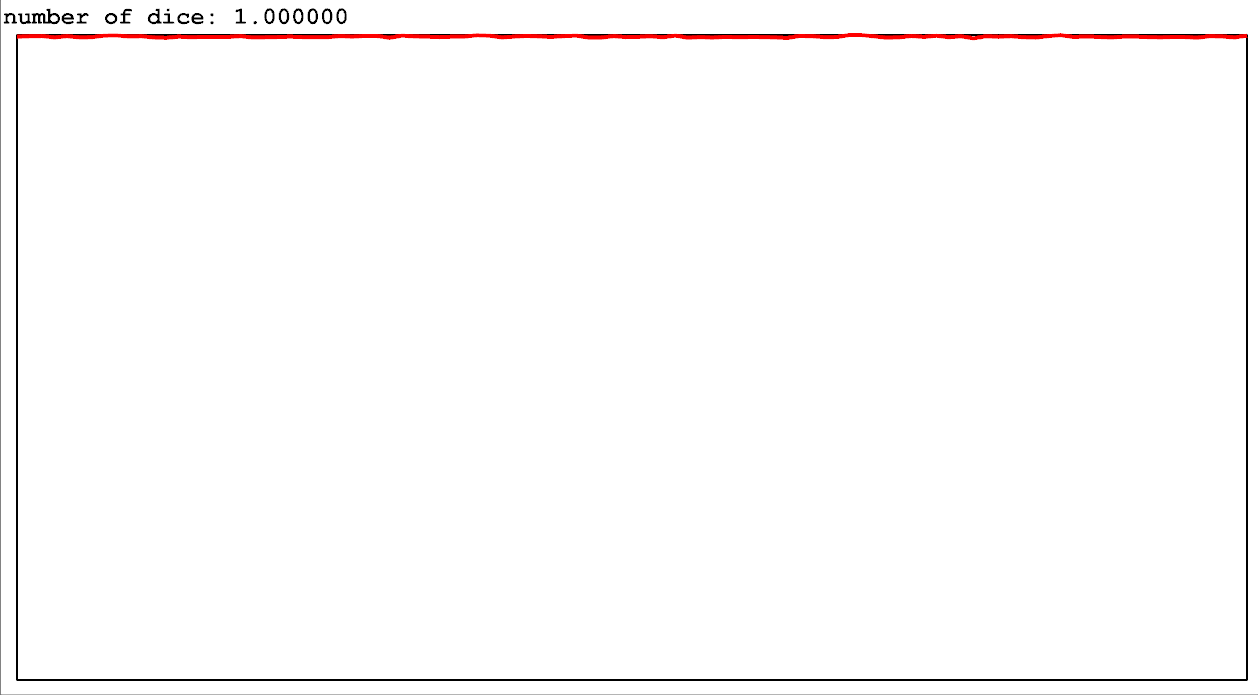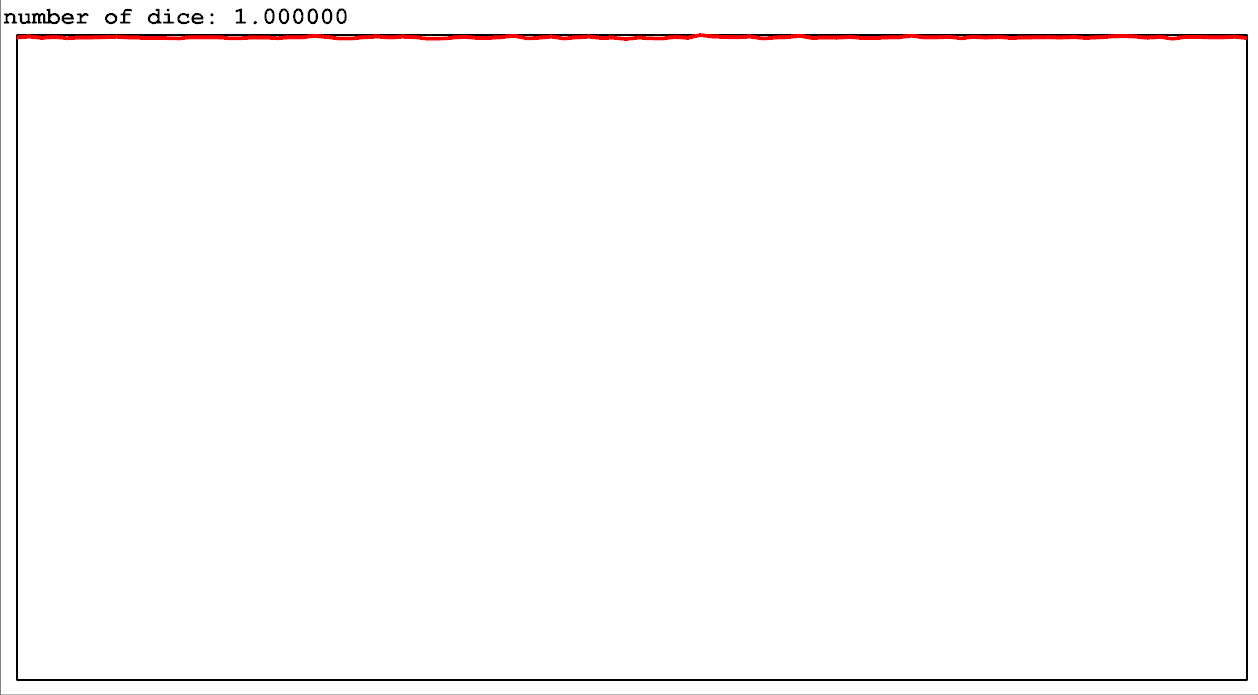# How to roll half a dice

Lets say you’re designing a game where, in order to determine the success of an action, you roll a d20 (a dice with 20 sides). Even if you’re making a digital game without dice, this can be a useful tool for contextualizing probabilities. Most of us have rolled dice before, so most of us intuitively know how this kind of random chance feels.

Now lets say you want to make a character “lucky”. Instead of rolling one d20, you roll two and take the highest result. You now have a twice(ish) as much chance of rolling a 20! But you have 20x less chance of rolling a 1 (the only way to roll a 1 now is to have both dice roll a 1, which is a 1 in 400 chance!) This character is certainly very lucky and he will almost never fail at what he’s trying to do.

But how do you get something in between? Maybe you want a character to be slightly lucky, but not so lucky that they essentially never roll a 1. You want the odds stacked in their favor, but still want them to have reasonable chances of bad rolls. This was a game design question I needed to figure out the other day. So I was curious, what if you could roll “1 and a half dice” instead of 2? What would that mean and what would the consequences look like?

So lets check the existing mathematical literature on the topic. Here is a paper about how to flip “half a coin”. The main construct here is that flipping 2 “half-coins” matches the results you’d expect from flipping one coin (50% chance of 1 heads and 50% chance of 1 tails), similar to how flipping “2 coins twice” is the same thing as flipping “4 coins” when counting the number of heads and tails in the results. So what does a half coin look like? Well it’s an infinitely sided coin where the chance of hitting specific sides can have negative probabilities. Which is… uh…

How does a negative probability even make sense? It basically doesn’t, outside of weird theoretical math stuff and maybe quantum mechanics. It’s cool that you can actually do this with some weird mathematical constructs, but this doesn’t actually help you in any way whatsoever when dealing with game design. So lets back up a bit and try to figure this out more… uh… comprehensibly.

Now my initial thought was that “1.5d20” could mean, 50% chance of rolling 1d20, 50% chance of rolling 2d20. Basically, flip a coin to determine if you get “luck” this roll or not. (Some games, like DnD, refer to additional rolls like this as “Advantage”). And this pretty much does exactly what I want. This generalizes out really nicely to any kind of fractional dice rolls, 1.1d20, 7.8d20, you name it. Hell you can even extend it to negative rolls if you just consider “-2d20” as meaning roll 2 and take the lowest.

But I was not entirely satisfied here. Something about this felt like it probably wasn’t the “mathematically correct” way to do this. Also, implementing this in code meant a for loop where you take a whole bunch of random numbers and return the max. What if you want to do insane numbers, like 1000000d20? Getting the max of a million numbers is much more expensive than getting the max of a couple of numbers. My motivation here was less about performance though, and more about wanting to just find a closed form formula that gives the same distribution as rolling multiple dice, in the hopes it maps to fractional values in a more mathematically satisfying way.

So I set out to try and figure this out. Quick piece of relevant information here: To calculate random integers in a range with code, you first grab a random real number between 0.0 and 1.0 (exclusive, you can get exactly 0 but you can’t get exactly 1), then map that number to the range you want (to map it to the range 1 to 20 you multiply by 20 and add 1, you now have a number between 1 and 21 (but never exactly 21), you then cut off the decimal points to get an integer between 1 and 20)

Now you should be able to run that initial random number (between 0 and 1) through a function first (I’ll call this a “random result modifier function”), then when you map it to “1–20” you end up with different probabilities for each number. What range you map this to doesn’t actually matter that much here since the shape of the distributions will look the same no matter how you map it (just chunkier), so I’m just going to work with these continuous 0 to 1 ranges instead for now.

Let’s take a quick look at what these distributions look like as histograms. This is a quick app I wrote that simulates a ton of continuous dice rolls and then displays the results as a histogram. No units shown since, again, its really just the shape that matters (but left side is 0, right side is 1, and the area under the curve is always equal to 1 (probabilities, yo!)). Notice that when you roll one die, the curve is a flat line (equal probabilities for every option). When you roll 2 dice, you get an increasing line (higher chance of rolling higher numbers).

Now the thing you should notice is that this is basically just a simple polynomial each time! At 1 dice, the graph looks like x⁰, at 2 dice it looks like x¹, at 3 dice it looks like x² and so on. A simple exponential formula, which does have a natural extension into fractional numbers! So lets just try that shall we. Lets just take our original random number, and raise it to the power n-1 (where n is the number of dice to roll). Uh…function: roll one continuous dice, apply f(x) = x^(n-1) to it

Yeah that didn’t quite work and its cause the function that represents the distribution we want doesn’t map to the function we use to modify our original random number directly like that. There’s apparently some calculus relationship here, which makes sense since the area under the curve is a relevant value here. There’s also an inverse relationship as well, which makes sense since if our function likes to pull values towards 0 (such as x² does within this range), that means that lower values are going to be way more common. There’s probably some rigorous mathematical way to transform a desired distribution function to a random result modifier function, but I just decided to trial and error it instead. If we raise it to the power of 1/n instead, we get what we want here.function: roll one continuous dice, apply f(x) = x^(1/n) to it

And now we can test this function with fractional dice!function: roll one continuous dice, apply f(x) = x^(1/n) to it

The main issue here is that rolling 1.1 dice tends to really cut off the chances of getting low rolls, much more than “10% luck” would imply. And it feels weird that the function in between a flat line and a straight line would be some weird sqrt-ish looking curve. While I think this is probably the best result we can get for “mathematically accurate” partial dice rolls, I also think that it kind of fails at what you’d want from a game design perspective.

So uh, lets go back and check the original thought I had for partial dice rolls. 1.5 dice rolls means 50% chance of one dice, 50% chance of two. The probability distributions for this method look like this:function: take p = the fractional portion of n. 1-p chance of floor(n) dice rolls, p chance of ceil(n) dice rolls

Oh that looks way nicer actually. The in-between values actually look like the functions that would end up in-between the integer values. We can also semi-closed form it considering that the power version agrees with this when number of dice is an integer, so we can still use that once we know how many “integer rolls” we want. One roll to determine how many rolls to use, then one roll, shoved through a power function, to get a result. Sweet! This method also generalizes pretty nicely to other forms of dice rolls too (such as adding up the results of rolling multiple dice).

It turns out this is yet another one of those things where the “mathematically correct” way to do something isn’t really the correct way to do something in the context of game design. I may have wasted my time exploring this stuff so much. But at least now I know!

So, to answer the question raised by the title of this article, How do you roll half a dice? Simple. Flip a coin, if heads roll a dice. If tails, don’t.

Programmer & Game Designer

## More from Tyler Glaiel

Programmer & Game Designer